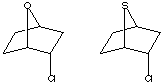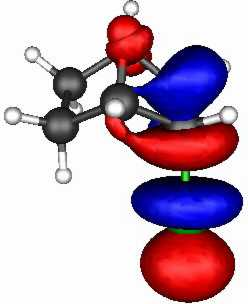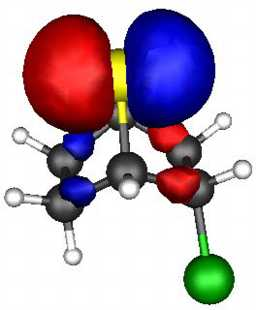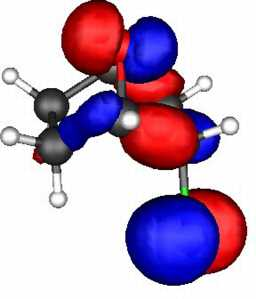S vs O as a Neighbouring Group

In a first order analysis, a nucleophilic/electrohilic interaction can be approximated by the interaction of two key molecular orbitals; the HOMO (the nucleophile) donating a a pair of electrons to the empty LUMO (the electrophile). This interaction depends on:
• The energy gap between these two orbitals. The smaller this gap, the better the interaction. Specifically, the higher the energy of the HOMO (i.e the less negative) the better a nucleophile it represents, whilst the lower the energy of the LUMO (i.e. less positive or more negative) the better the electrophile, and the combination of these two is the HOMO-LUMO gap.
• The spatial overlap of the HOMO with the LUMO.
These two effects can be probed by a molecular orbital calculation on the two reactants, for which acetolysis for the S system shown below is observed to be 109 faster than for the O analogue.The results (ab initio 3-21G* level) are as follows.

• S bicyclic: HOMO-LUMO gap = 0.546 Hartree.
• O bicyclic: HOMO-LUMO gap = 0.606 Hartree.
The difference amounts to 157 kJ (1 Hartree = 2624 kJ). The spatial overlap can be seen below. On both counts, S wins over O.
S bicyclic O bicyclic
LUMOHOMOProduct Stability

One can test the above (reactant based) conclusions by calculating the energy of the carbocation resulting from elimination of Cl-.
SO
Thus the energy to create a cation from the O system is +980 kJ whereas that for the S system is +898 kJ (AM1 calculations). Solvation and other factors not withstanding, this difference of 82 kJ abundantly explains the difference in solvation rates. Notice in particular that ring strain prevents the O system from closing to form an oxonium ion, whereas the larger more flexible S system does indeed so close.